Question
an ieee test power system has 30 buses, among which 7 are generator buses. determine the number of slack bus , pv bus and pq bus and the dimension of jacobian matrix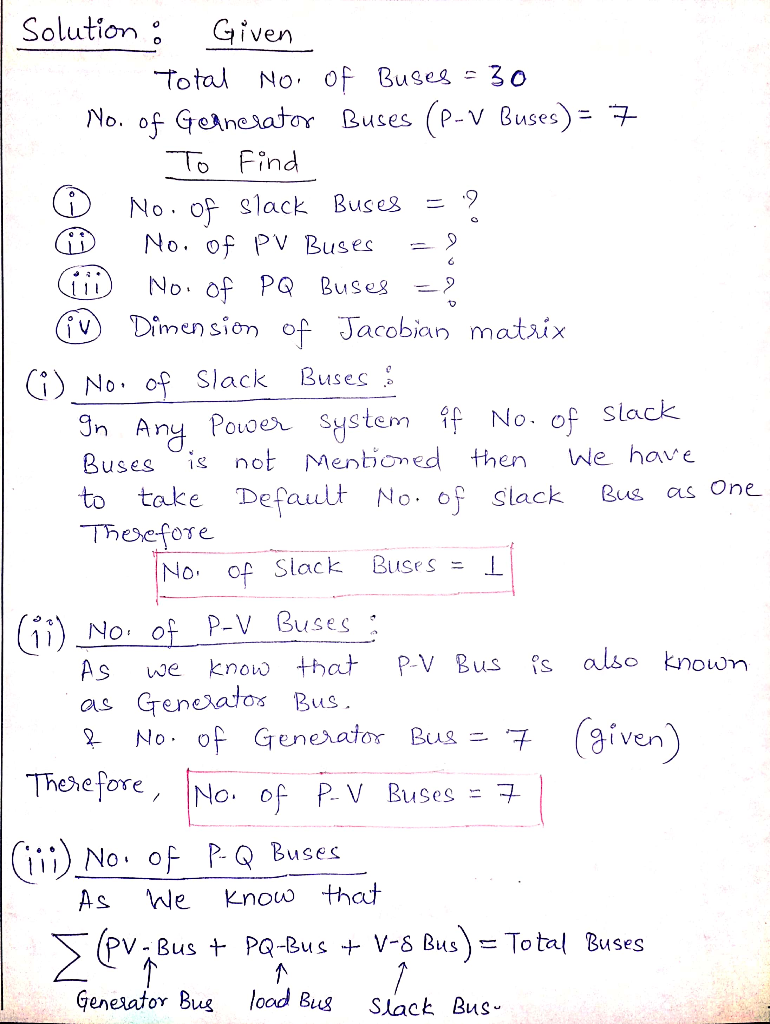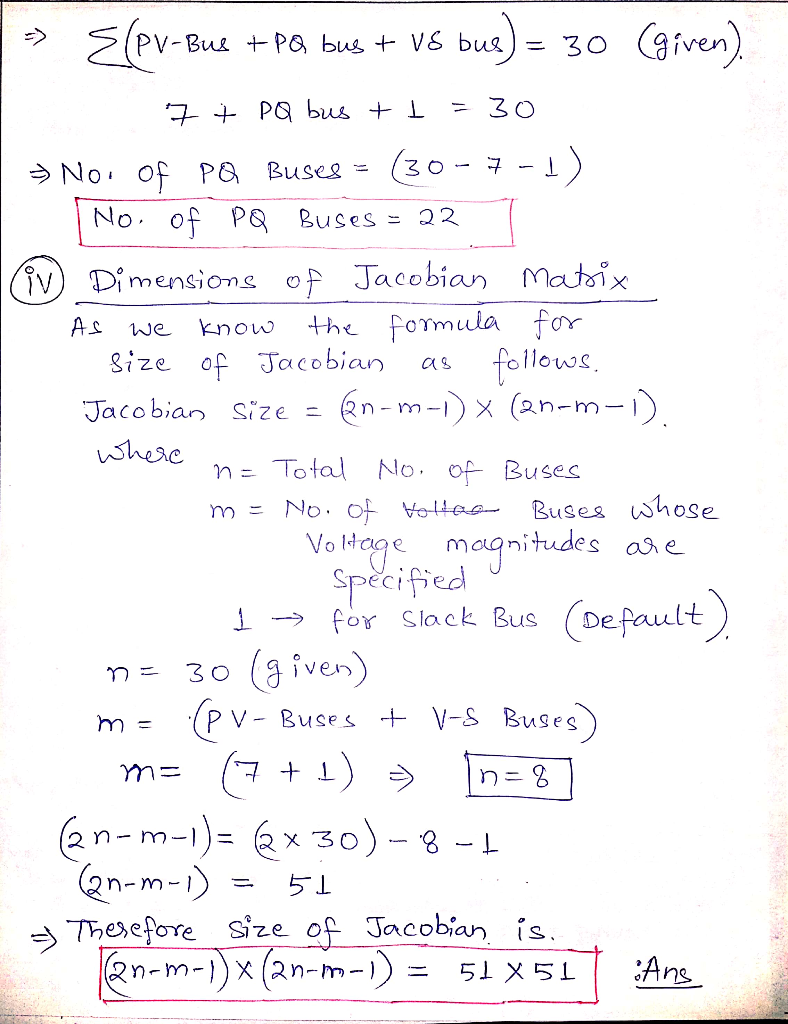​​​​​​

#### Earn Coins

Coins can be redeemed for fabulous gifts.

Similar Homework Help Questions
• ### The single-line diagram of a three-phase live-bus power system is shown in Fig. 1. All lines...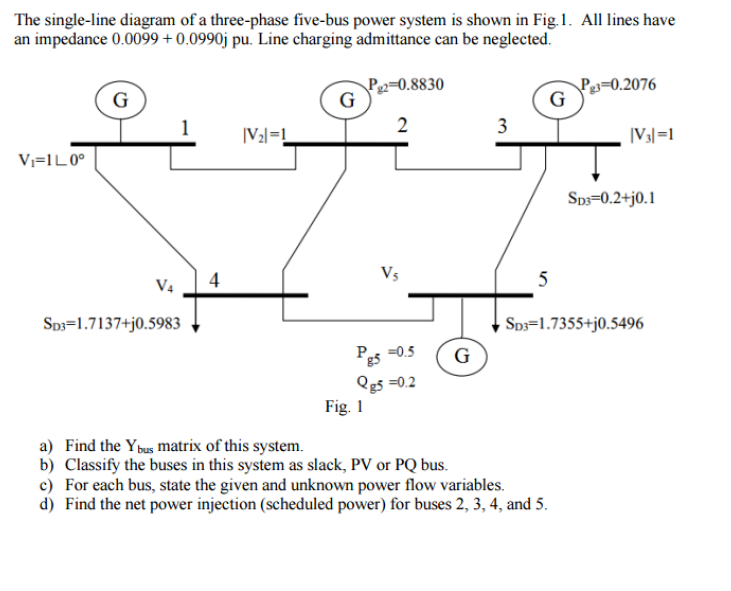The single-line diagram of a three-phase live-bus power system is shown in Fig. 1. All lines have an impedance 0.0099 + 0.0990j pu. Line charging admittance can be neglected. a) Find the Ybus matrix of this system. b) Classify the buses in this system as slack, PV or PQ bus. c) For each bus, state the given and unknown power how variables. d) Find the net power Injection (scheduled power) for buses 2, 3, 4, and 5.

• ### The single-line diagram of a three-phase five-bus power system is shown in Fig.1. All lines have ...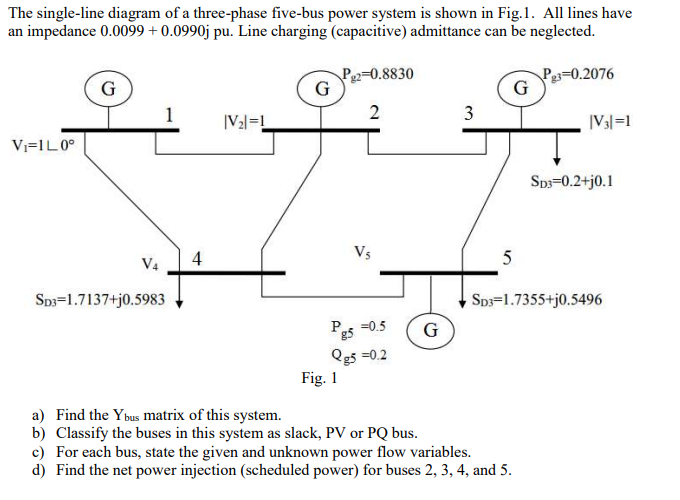The single-line diagram of a three-phase five-bus power system is shown in Fig.1. All lines have an impedance 0.0099+0.0990j pu. Line charging (capacitive) admittance can be neglected. 2-0.8830 30.2076 SD3-0.2+j0.1 SD3-1.7137+j0.5983 Sos-1.7355+j0.5496 Pe5 -0.5 G Qg5 -0.2 Fig. 1 a) Find the Ybus matrix of this system. b) Classify the buses in this system as slack, PV or PQ bus c) For each bus, state the given and unknown power flow variables. d) Find the net power injection (scheduled power)...

• ### Consider the following power system consisting of three buses, All of the values are given in pu....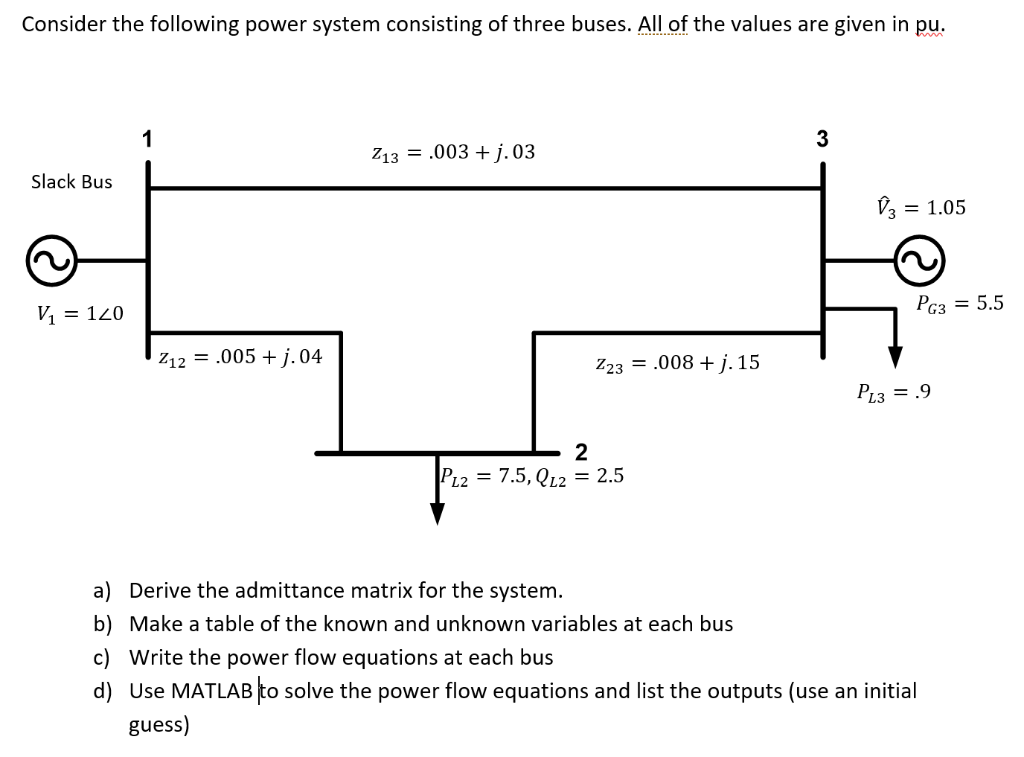Consider the following power system consisting of three buses, All of the values are given in pu. 3 Z13003+.03 Slack Bus = 1.05 Рез-5.5 И 120 1205j.04 Z23 = .008 + j, 15 P13.9 2 PL2 = 7.5, QL2 = 2.5 a) b) c) d) Derive the admittance matrix for the system. Make a table of the known and unknown variables at each bus Write the power flow equations at each bus Use MATLAB to solve the power flow equations...

• ### Problem #1: Take a two-bus system. Bus #1 is represented as an infinite bus with a constant voltage of 120 per unit. Bus #2 is represented as a load / PQ bus with a constant complex power draw (consu...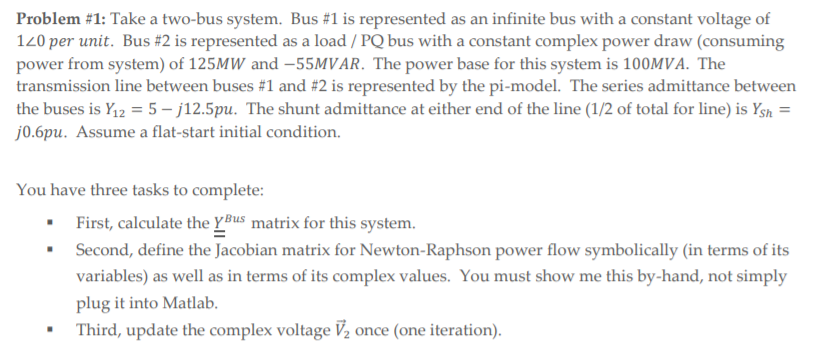Problem #1: Take a two-bus system. Bus #1 is represented as an infinite bus with a constant voltage of 120 per unit. Bus #2 is represented as a load / PQ bus with a constant complex power draw (consuming power from system) of 125MW and-55MVAR. The power base for this system is 100MVA. The transmission line between buses #1 and #2 is represented by the pi-model. The series admittance between the buses is Y12-5-12.5pu. The shunt admittance at either end...

• ### Consider the two bus system in the figure. The line has series impedance of 0.01 + j0.05 pu and a...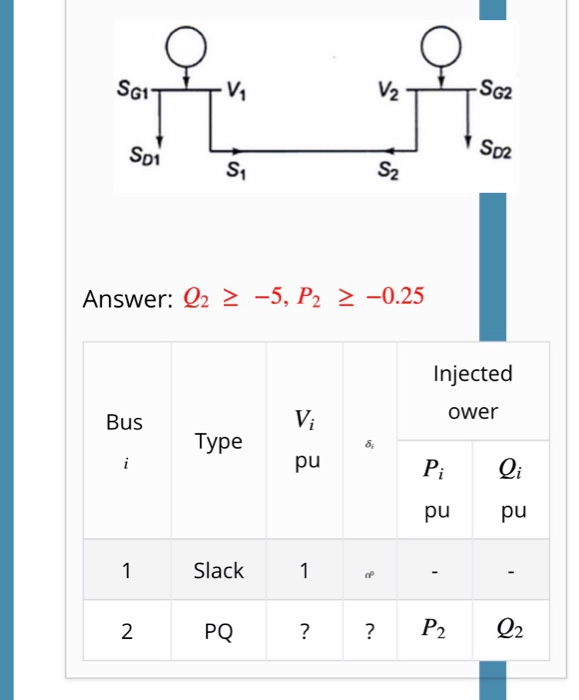Consider the two bus system in the figure. The line has series impedance of 0.01 + j0.05 pu and a total shunt admittance of j0.01 pu. Assume Pi-model for the line. The specified quantities at the two buses are given in the table below. Determine the range of P_2 and Q_2 for which the solution of exists. Use the power flow equation (7). SG1 SD1 S02 S1 S2 Answer: 02 2 -5, P2 2 -0.25 Injected ower Bus Type pu...

• ### Consider the single line diagram of a 3-bus power system shown in Figure 2. Slack bus...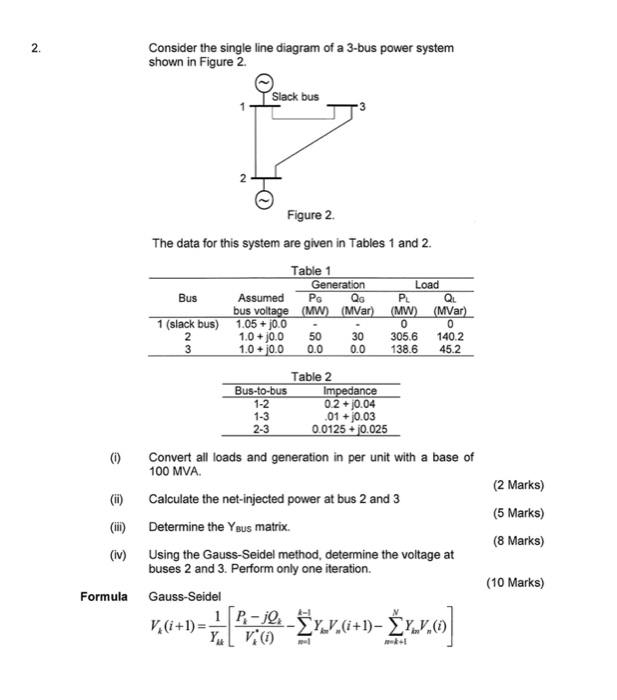Consider the single line diagram of a 3-bus power system shown in Figure 2. Slack bus 3 Figure 2. The data for this system are given in Tables 1 and 2. Bus Table 1 Generation Load Assumed PG QGPLQL bus voltage (MW) (MVar) (MW) (MVar) 1.05 +10.0 - - 1.0 + 0.0 50 30 305.6 140.2 1.0 +0.0 0.0 0.0 138.6 45.2 slack bus) Table 2 Bus-to-bus Impedance 0.2 + j0.04 .01 +0.03 2.3 0.0125 + j0.025 (0) Convert all...

• ### Question 1: A single line diagram of a three-bus power system is shown in Fig 1....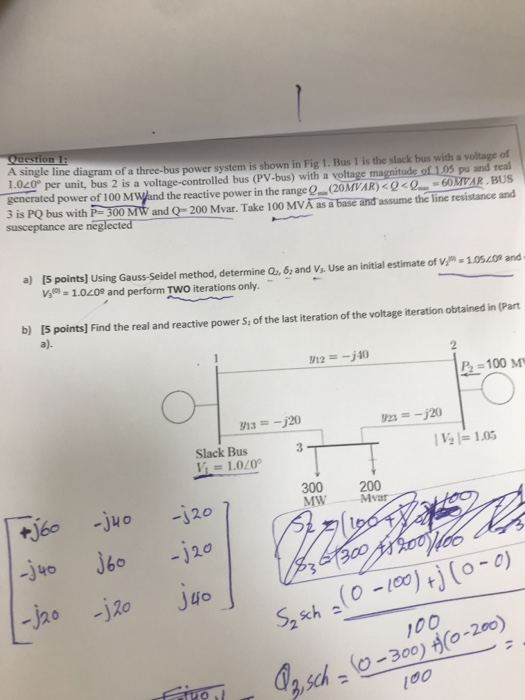Question 1: A single line diagram of a three-bus power system is shown in Fig 1. Bus 1 is the slack bus with a voltage of 1.020 per unit, bus 2 is a voltage-controlled bus (PV-bus) with a voltage magnitude of 1.05 pu and real generated power of 1 00 MWand the reactive power in the range Q.(20MVAR) < Q<Q-60M¥AR .BUS 3 is PQ bus with P 300 MW and Q= 200 Mvar. Take 100 MVÅ susceptance are neglected as...

• ### Q2. (40) Eig. 1 shows the one-line diagram of a simple three-bus power system with generation at bus 1. The magnitude of voltage at bus 1 is adjusted to 1.05 pu. The scheduled loads at buses 2 and 3...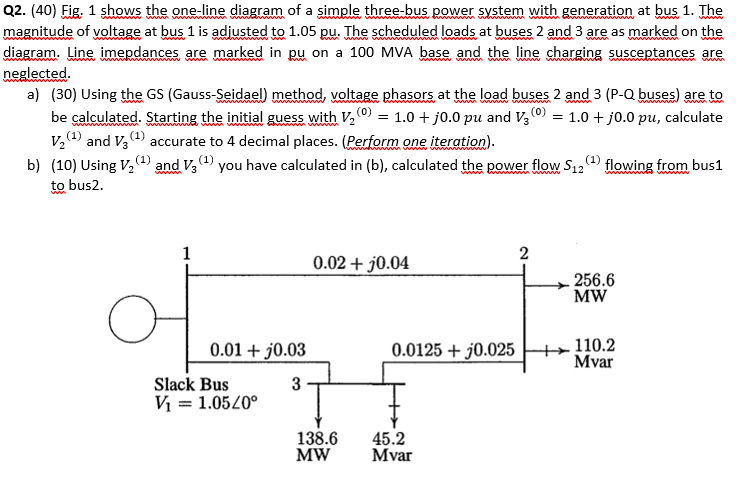Q2. (40) Eig. 1 shows the one-line diagram of a simple three-bus power system with generation at bus 1. The magnitude of voltage at bus 1 is adjusted to 1.05 pu. The scheduled loads at buses 2 and 3 are as marked on the diagram. Line imepdances are marked in pu on a 100 MVA base and the line charging susceptances are neglected a) (30) Using the GS (Gauss-Seidael) method, voltage phasors at the load buses 2 and 3 (P-Q...

• ### BUS BUS 2 Line 1 Line 2 Line 3 Line 4 BUS 3 BUS 4 4x4 bus admittance matrix for the above power system Y Generator acti...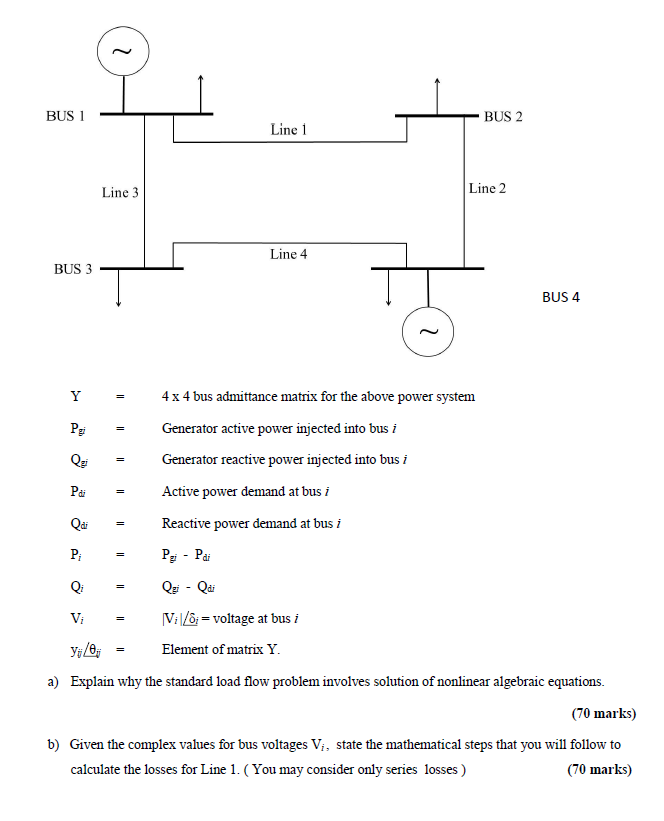BUS BUS 2 Line 1 Line 2 Line 3 Line 4 BUS 3 BUS 4 4x4 bus admittance matrix for the above power system Y Generator active power injected into bus i Generator reactive power injected into bus i Pa Active power demand at bus i Qa Reactive power demand at bus i P Pai IVivoltage at bus / Vi Element of matrix Y a) Explain why the standard load flow problem involves solution of nonlinear algebraic equations. (70 marks)...

• ### ELEG 330 Power System Analysis Problem 2: A generator is feeding 30 MW to an infinite...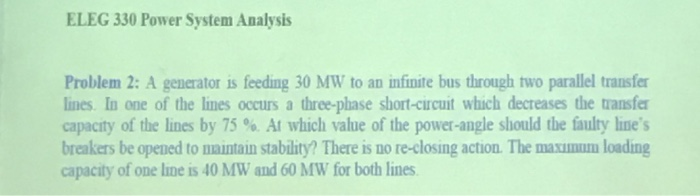ELEG 330 Power System Analysis Problem 2: A generator is feeding 30 MW to an infinite bus through two parallel transfer lines. In one of the lines occurs a three-phase short-circuit which decreases the transfer capacity of the lines by 75 %. At which value of the power-angle should the faulty line's breakers be opened to maintain stablity? There is no re-closing action. The maxmom loading capacity of one line is 40 MW and 60 MW for both lines